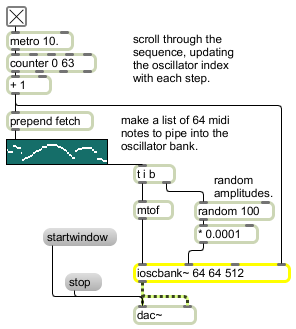# ioscbank~

Interpolating oscillator bank

## Description

ioscbank~ is an interpolated oscillator bank with signal inputs to set oscillator frequency and magnitude.

## Arguments

Name Type Opt Description
number-of-oscillators int opt The number of oscillators. The default is 1.
frequency-smoothing-factor (samples) int opt The number of samples across which frequency smoothing is done.
amplitude-smoothing-factor (samples) int opt The number of samples across which amplitude smoothing is done.

## Messages

 int inlet-specific-parameter-settings [int] Performs the same function as float in any inlet (see signal and float messages). float inlet-specific-parameter-settings [float] In left inlet: Sets the frequency of the oscillator whose index is currently referenced to the current floating-point value of the signal. The default value is 0. In 2nd inlet: Sets the magnitude (amplitude) of the oscillator whose index is currently referenced. In 3rd inlet: If framesync is enabled using the framesync 1 message, a signal in the range 0-1.0 sets the phase of the oscillator currently being referenced. In 4th inlet: Sets the index of the oscillator currently being referenced. clear The word clear sets the frequency of all oscillators to zero and zeros all amplitudes. copybuf buffer-object-name [symbol]offset (samples) [int] In left inlet: The word copybuf, followed by a symbol that specifies a buffer, copies 4096 samples from the buffer into the ioscbank~ object's internal wavetable. An optional second integer argument specifies the position in the buffer at which samples are loaded (offset). framesync behavioral-flag (0 or nonzero) [int] The word framesync, followed by a non-zero number, enables frame synchronous operation. When frame synchronous operation is enabled, a given index's values will only change or begin their interpolated ramps to the next value when the index input signal is 0 (or once per n sample frame). Otherwise, a given index's values will change or begin their interpolated ramps to the next value when the index input signal is equal to that index. The default is off. freqsmooth frequency-smoothing-factor (samples) [int] The word freqsmooth, followed by a number, sets the number of samples across which frequency smoothing is done. The default is 1 (no smoothing). magsmooth amplitude-smoothing-factor (samples) [int] The word magsmooth, followed by a number, sets the number of samples across which magnitude (amplitude) smoothing is done on an oscillator. The default is 0 (no amplitude smoothing). set frequency-amplitude-pairs (floats) [list] The word set, followed by pairs of floating-point values, sets the frequency and amplitude of an oscillator in the oscillator bank. A list of n pairs will set the first n oscillators in the ioscbank~ object and zero the amplitude of all others. signal Performs the same function(s) as a float corresponding to each inlet. silence The word silence zeros the amplitude of all the oscillators. size number-of-oscillators [int] The word size, followed by a number, sets the number of oscillators. The default is 64.

## Output

signal: A waveform consisting of the sum of the specified frequencies and amplitudes.

## Examplesioscbank~ lets you sound multiple interpolated oscillators with one object